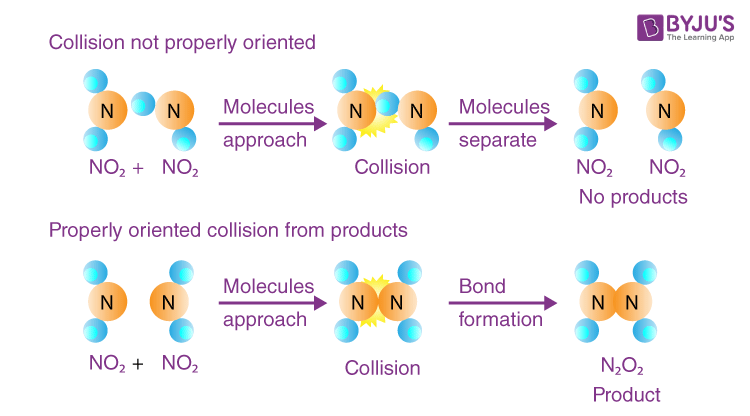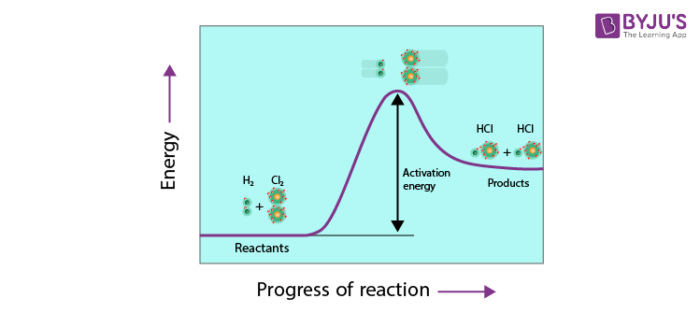# The Collision Theory Of Chemical Reactions

The study of Collision theory of chemical reactions and their kinetics has made great developments that play a pivotal role in today’s fast-paced world. Be it the packaged drinking water, the water bottles or the steel manufacturing plant or the fastest motor vehicles or the synthetically designed biological implants, some way or the other there is a chemical reaction associated with them.

In order to get a better insight into these chemical reactions, Max Trautz and William Lewis in 1916-1918 developed Collision Theory of Chemical Reactions based on the kinetic theory of gases. All of us have heard about the kinetic theory of gases. It explains the behaviour of gases by considering them many particles, molecules or atoms that are constantly moving in random directions.

## Collision Theory of Chemical Reactions

According to the collision theory, “the molecules of reactants are assumed to be hard spheres and the reactions are assumed to occur only when these spheres (molecules) collide with each other”. So it was important to quantify the number of collisions occurring in order to form products so that we can have a clear picture of the reaction, and hence came the term collision frequency.

Collision Frequency can be understood as the number of collisions per second per unit volume of the reacting mixture. It is generally denoted by Z.

Let’s consider the following bimolecular elementary reaction:

P + Q → Product

Now as per the collision theory, the rate of the above reaction can be given by:

Rate=ZPQ ρ e−Ea/RT

Where:

• ZPQ = collision frequency of reactants P and Q
• E= Activation Energy
• R = Universal Gas Constant
• T = Temperature in absolute scale
• ρ = is the steric factor

One more parameter that affected the rates of chemical reactions significantly is activation energy (Ea). The term activation energy was given by Arrhenius, it is the minimum amount of energy that the reactants must possess in order to form a product during a chemical reaction.

As per the Arrhenius Equation, all the molecules that have energy greater than or equal to activation energy will collide to form products. But this postulate was not true for all the reactions. A significant amount of deviation was observed in reactions involving complex molecules.

Some molecules having sufficient energy (activation energy) did not collide to form the product. Only a few of them made effective collisions, collisions that led to the formation of products. The scientists found that the kinetic energy of the molecules is not the only parameter that governs the reaction.

They concluded that only those molecules that have the threshold energy (activation energy) and proper orientation during the collision will form products. They introduced a probability factor P to account for the effective collisions.

So now the rate of a reaction is given by

$$\begin{array}{l} Rate = PZ_{PQ} e^{\frac{-E_a}{RT}}…………………………..(2)\end{array}$$In a nutshell, we can say that the activation energy and proper orientation of the reacting molecules together determine the condition for a collision that will result in the formation of products that is an effective collision. So in collision theory, both activation energy and effective collision govern the rate of a reaction.## Activation Energy

The activation energy is the minimum amount of energy needed by the reacting particles in any particular reaction for that reaction to take place. Unless particles collide with sufficient energy to supply the activation energy they simply don’t react. The activation energy must be supplied before a reaction can take place.

To start a chemical reaction chemical bonds need to be broken in the reactants, which requires energy. The energy needed to start the reaction is known as activation energy. Sometimes the activation energy is low so that the reaction can start at room temperature without heating.## Arrhenius Equation

Temperature has a very significant effect on the rate of chemical reaction. In general the rate of Chemical reactions increases with the rise in temperature. In most cases, the reaction rate becomes double while in a few cases, it may be even more than double for every 10 K rise in temperature. The value of the temperature coefficient which is the ratio of the rate constants  at the two different temperatures generally lies between 2 and 3.

Temperature coefficient= (rate constant at 310K)/(rate constant at 300K )= 2 to 3

Arrhenius developed a mathematical relationship between temperature and the rate constant. The temperature dependence of the rate constant, k is expressed algebraically as follows.

K  ∝  e-Ea/RT

K = A e-Ea/RT

This equation is Known as the Arrhenius equation.

Here A is known as frequency factor. Ea is the activation energy , R is gas constant and T is the absolute temperature.

For detail explanation of Arrhenius equation visit ⇨ Arrhenius equation

Q1

### 1. How does collision theory work?

According to the collision theory, the molecules of reactants are assumed to be hard spheres and the reactions are assumed to occur only when these spheres (molecules) collide with each other. It is not necessary that all the collisions may lead to the products, the activation energy and proper orientation of the reacting molecules together determine the condition for a collision that will result in the formation of products.

Q2

### 2. What is the difference between effective and ineffective collision?

The reacting species taking part in a chemical reaction can give products only in case they come in contact with each other or they collide with each other. It is not necessary that all the collisions may lead to the products. The collisions among the reacting species which result in the product are known as effective collisions.

Q3

### 3. Is Arrhenius equation only for first order reaction?

The Arrhenius equation is an expression that provides a relationship between the rate constant , the absolute temperature, and the A factor (also known as the pre-exponential factor; can be visualized as the frequency of correctly oriented collisions between reactant particles). It provides insight into the dependence of reaction rates on the absolute temperature. So it can be applicable to any order of reaction.

Q4

### 4. What is known as activation energy?

Activation energy is defined as the extra amount of energy which has to be supplied to the reacting species in their normal energy state to make their energy equal to threshold energy.

Q5

### 5. Where is the Arrhenius equation used?

The Arrhenius equation used to determine the effect of a change of temperature on the rate constant. Arrhenius developed a mathematical relationship between temperature and the rate constant.

Test Your Knowledge On The Collision Theory Of Chemical Reactions!